# Fluid

Continuum mechanics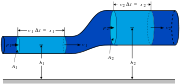Conservation of mass
Conservation of momentum
Navier–Stokes equations
Tensors

A fluid is defined as a substance that continually deforms (flows) under an applied shear stress regardless of the magnitude of the applied stress. It is a subset of the phases of matter and includes liquids, gases, plasmas and, to some extent, plastic solids. The study of fluids is fluid mechanics, which is subdivided into fluid dynamics and fluid statics, depending on whether the fluid is in motion or not.

Fluids are also divided into liquids and gases. Liquids form a free surface (that is, a surface not created by their container) while gases do not. The distinction between solids and fluids is not so obvious. The distinction is made by evaluating the viscosity of the matter: for example silly putty can be considered either a solid or a fluid, depending on the time period over which it is observed.

Fluids share the properties of not resisting deformation and the ability to flow (also described as their ability to take on the shape of their containers). These properties are typically a function of their inability to support a shear stress in static equilibrium. While in a solid, stress is a function of strain, in a fluid, stress is a function of rate of strain. A consequence of this behavior is Pascal's law which entails the important role of pressure in characterizing a fluid's state.

Based on how the stress depends on the rate of strain and its derivatives, fluids can be characterized as:

• Newtonian fluids : where stress is directly proportional to rate of strain, and
• Non-Newtonian fluids : where stress is proportional to rate of strain, its higher powers and derivatives (basically everything other than Newtonian fluid).

The behavior of fluids can be described by a set of partial differential equations, which are based on the conservation of mass, linear and angular momentum (Navier-Stokes equations) and energy.

The properties of fluids have found numerous applications in hydraulics, which utilizes fluids to transmit force at a distance from the control point. Hydraulic earth-moving machinery and hydraulic control systems in aircraft are well-known examples. Knowledge of fluids is essential for the design and construction of hydraulic structures, like bridges, dams, canals, channels, and levees; this is the field of hydraulic engineering. Understanding the fluid properties of blood is essential for designing biomechanical and prosthetic devices. The emerging field of fluidics uses the properties of fluids to build fluid-based digital and analog circuits for control devices.

## Newtonian fluids

A Newtonian fluid (named for Isaac Newton) is a fluid that flows like water—its shear stress is linearly proportional to the velocity gradient in the direction perpendicular to the plane of shear. The constant of proportionality is known as the viscosity.

A simple equation to describe Newtonian fluid behavior is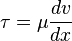$\tau=\mu\frac{dv}{dx}$

where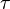$\tau$ is the shear stress exerted by the fluid ("drag") [Pa]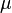$\mu$ is the fluid viscosity - a constant of proportionality [Pa·s]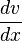$\frac{dv}{dx}$ is the velocity gradient perpendicular to the direction of shear [s−1]

In common terms, this means the fluid continues to flow, regardless of the forces acting on it. For example, water is Newtonian, because it continues to exemplify fluid properties no matter how fast it is stirred or mixed. Contrast this with a non-Newtonian fluid, in which stirring can leave a "hole" behind (that gradually fills up over time - this behaviour is seen in materials such as pudding, oobleck, or, to a less rigorous extent, sand), or cause the fluid to become thinner, the drop in viscosity causing it to flow more (this is seen in non-drip paints).

For a Newtonian fluid, the viscosity, by definition, depends only on temperature and pressure (and also the chemical composition of the fluid if the fluid is not a pure substance), not on the forces acting upon it.

If the fluid is incompressible and viscosity is constant across the fluid, the equation governing the shear stress, in the Cartesian coordinate system, is$\tau_{ij}=\mu\left(\frac{\partial v_i}{\partial x_j}+\frac{\partial v_j}{\partial x_i} \right)$

where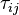$\tau_{ij}$ is the shear stress on the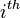$i^{th}$ face of a fluid element in the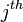$j^{th}$ direction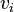$v_i$ is the velocity in the$i^{th}$ direction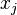$x_j$ is the$j^{th}$ direction coordinate

If a fluid does not obey this relation, it is termed a non-Newtonian fluid, of which there are several types.

## Non-Newtonian fluids

A non-Newtonian fluid is a fluid in which the viscosity changes with the applied strain rate. As a result, non-Newtonian fluids may not have a well-defined viscosity.

Although the concept of viscosity is commonly used to characterize a material, it can be inadequate to describe the mechanical behavior of a substance, particularly non-Newtonian fluids. They are best studied through several other rheological properties which relate the relations between the stress and strain tensors under many different flow conditions, such as oscillatory shear, or extensional flow which are measured using different devices or rheometers. The rheological properties are better studied using tensor-valued constitutive equations, which are common in the field of continuum mechanics.

### Common examples

An inexpensive, non-toxic sample of a non-Newtonian fluid can be made very easily by adding corn starch to a cup of water. Add the starch in small portions and stir it in slowly. When the suspension nears the critical concentration - becoming like single cream in consistency - the so called "shear thickening" property of this non-Newtonian fluid becomes apparent. The application of force - for example by stabbing the surface with a finger, or rapidly inverting the container holding it - leads to the fluid behaving like a solid rather than a liquid. More gentle treatment, such as slowly inserting a spoon, will leave it in its liquid state. Trying to jerk the spoon back out again, however, will trigger the return of the temporary solid state. A person moving quickly and/or applying sufficient force with his feet can literally walk across such a liquid (see video link below).

Shear thickening fluids of this sort are being researched for bullet resistant body armor, useful for their ability to absorb the energy of a high velocity projectile impact but remain soft and flexible while worn.

A familiar example of the opposite, a shear-thinning fluid, is paint: one wants the paint to flow readily off the brush when it is being applied to the surface being painted, but not to drip excessively.

### Types of Non-Newtonian fluidsPrincipal types of non-Newtonian fluids are given in the table below.

Type of fluid Behaviour Characteristics Examples
Plastic solids Perfectly plastic Strain does not result in opposing stress Ductile metals past the yield point
Bingham plastic Linear relationship between shear stress and rate of strain once threshold shear stress exceeded Mud, some colloids
Yield pseudo-plastic Pseudo-plastic above some threshold shear stress
Yield dilatent Dilatent above some threshold shear stress
Power-law fluids Pseudoplastic or "shear thinning" Apparent viscosity reducing with rate of shear Some colloids, clay, milk, gelatin, blood and liquid cement
Dilatant or "shear thickening" Apparent viscosity increasing with rate of shear Concentrated solution of sugar in water, suspensions of rice starch or corn starch
Viscoelastic - having both viscous and elastic properties Maxwell material "Series" linear combination of elastic and viscous effects metals, composite materials
Oldroyd-B fluid Linear combination of Maxwell and Newtonian behaviour Bitumen, dough, nylon, and Silly Putty
Kelvin material "Parallel" linear combination of elastic and viscous effects
Anelastic Material returns to a well-defined "rest shape"
Time-dependent viscosity Rheopectic Apparent viscosity increases with duration of stress Some lubricants
Thixotropic Apparent viscosity decreases with duration of stress Non-drip paints and tomato ketchup and most honey varieties.
Generalized Newtonian fluids Stress depends on normal and shear strain rates and also the presuure applied on it Blood, Custard

## SuperfluidsHelium II will "creep" along surfaces in order to find its own level - after a short while, the levels in the two containers will equalize. The Rollin film also covers the interior of the larger container; if it were not sealed, the helium II would creep out and escape.

Superfluidity is a phase of matter characterized by the complete absence of viscosity. Thus superfluids, placed in a closed loop, can flow endlessly without friction. Superfluidity was discovered by Pyotr Leonidovich Kapitsa, John F. Allen, and Don Misener in 1937. The study of superfluidity is called quantum hydrodynamics. Phenomenological theory of superfluidity in helium-4 was created by Lev Landau, whereas Nikolay Bogoliubov first suggested simple microscopical theory.

### Background

Although the phenomenologies of the superfluid states of helium-4 and helium-3 are very similar, the microscopic details of the transitions are very different. Helium-4 atoms are bosons, and their superfluidity can be understood in terms of the Bose statistics that they obey. Specifically, the superfluidity of helium-4 can be regarded as a consequence of Bose-Einstein condensation in an interacting system. On the other hand, helium-3 atoms are fermions, and the superfluid transition in this system is described by a generalization of the BCS theory of superconductivity. In it, Cooper pairing takes place between atoms rather than electrons, and the attractive interaction between them is mediated by spin fluctuations rather than phonons. See fermion condensate. A unified description of superconductivity and superfluidity is possible in terms of gauge symmetry breaking.

Superfluids, such as supercooled helium-4, exhibit many unusual properties. A superfluid acts as if it is a mixture between a normal component, with all the properties associated with normal fluid, and a superfluid component. The superfluid component has zero viscosity, zero entropy, and infinite thermal conductivity. (It is thus impossible to set up a temperature gradient in a superfluid, much as it is impossible to set up a voltage difference in a superconductor.) One of the most spectacular results of these properties is known as the thermomechanical or fountain effect. If a capillary tube is placed in a bath of superfluid helium, and the tube is heated (even by shining a light on it), the superfluid helium will flow up through the tube and out the top (this is a result of the Clausius-Clapeyron relation). A second unusual effect is that superfluid helium can form a layer, a single atom thick, up the sides of any container it is placed in.

A more fundamental property than the disappearance of viscosity becomes visible if superfluid is placed in a rotating container. Instead of rotating uniformly with the container, the rotating state consists of quantized vortices. That is, when the container is rotated at speed below the first critical velocity (related to the quantum numbers for the element in question) the liquid remains perfectly stationary. Once the first critical velocity is reached, the superfluid instantaneously starts spinning at the critical speed. The speed is quantized - i.e. it can only spin at certain speeds.

### Applications

Recently in the field of chemistry, superfluid helium-4 has been successfully used in spectroscopic techniques, as a quantum solvent. Referred to as Superfluid Helium Droplet Spectroscopy (SHeDS), it is of great interest in studies of gas molecules, as a single molecule solvated in a superfluid medium allows a molecule to have effective rotational freedom - allowing it to behave exactly as it would in the gas phase.

Superfluids are also used in high precision devices such as gyroscopes, which allow the measurement of some theoretically predicted gravitational effects, for example see Gravity Probe B article.

### Recent discoveries

Physicists have recently been able to create a Fermionic condensate from pairs of ultra-cold fermionic atoms. Under certain conditions, fermion pairs form diatomic molecules and undergo Bose–Einstein condensation. At the other limit the fermions (most notably superconducting electrons) form Cooper pairs which also exhibit superfluidity. This recent work with ultra-cold atomic gases has allowed scientists to study the region in between these two extremes, known as the BEC-BCS crossover.

Additionally, supersolids may have also been discovered in 2004 by physicists at Penn State University. When helium-4 is cooled below about 200 mK under high pressures a fraction (~1%) of the solid appears to become superfluid .